Array Fractions (Montessori Math Lesson) | Montessori From The Heart

Understanding fractions is an incredibly important concept as it forms a basis for later higher math, chemistry, physics, and many practical life activities. When Adrian turned two, we started talking about fractions in the kitchen while cutting an orange in halves, or cutting a pizza into quarters. Such real life models aid a child in visualization of a complex mathematical concept, such as fractions. Over time, once the terminology and visual modeling is mastered, you can move into simple fraction math and fraction reduction or simplification. Fraction exploration is best started with fractioning a circle, and tactile Montessori materials will help develop a firm understanding of fractions.

Today, we are learning fractions by using a beautiful hand-made fractions material (buy here), which is laser cut for precision and can be made to order from sustainable alder wood or maple. Each set is coated with an acrylic laquer for durability and wipe-clean use. Each fraction slice on one side is engraved with the value of the fraction. A frame allows a child to build a circle and then pop it up and build an equivalent fraction for comparison.We pretend that the circle we are fractioning is a 🍕pizza pie. Place all fractions mixed up in a basket. After showing a child "one whole pizza” pick a ½ slice and say:

This says one- half or ½ or 1 cut into two. How many pieces do we need to make one whole pizza?" – “We need two pieces to make a whole – we need two one-halves.” Have the child find the other one-half.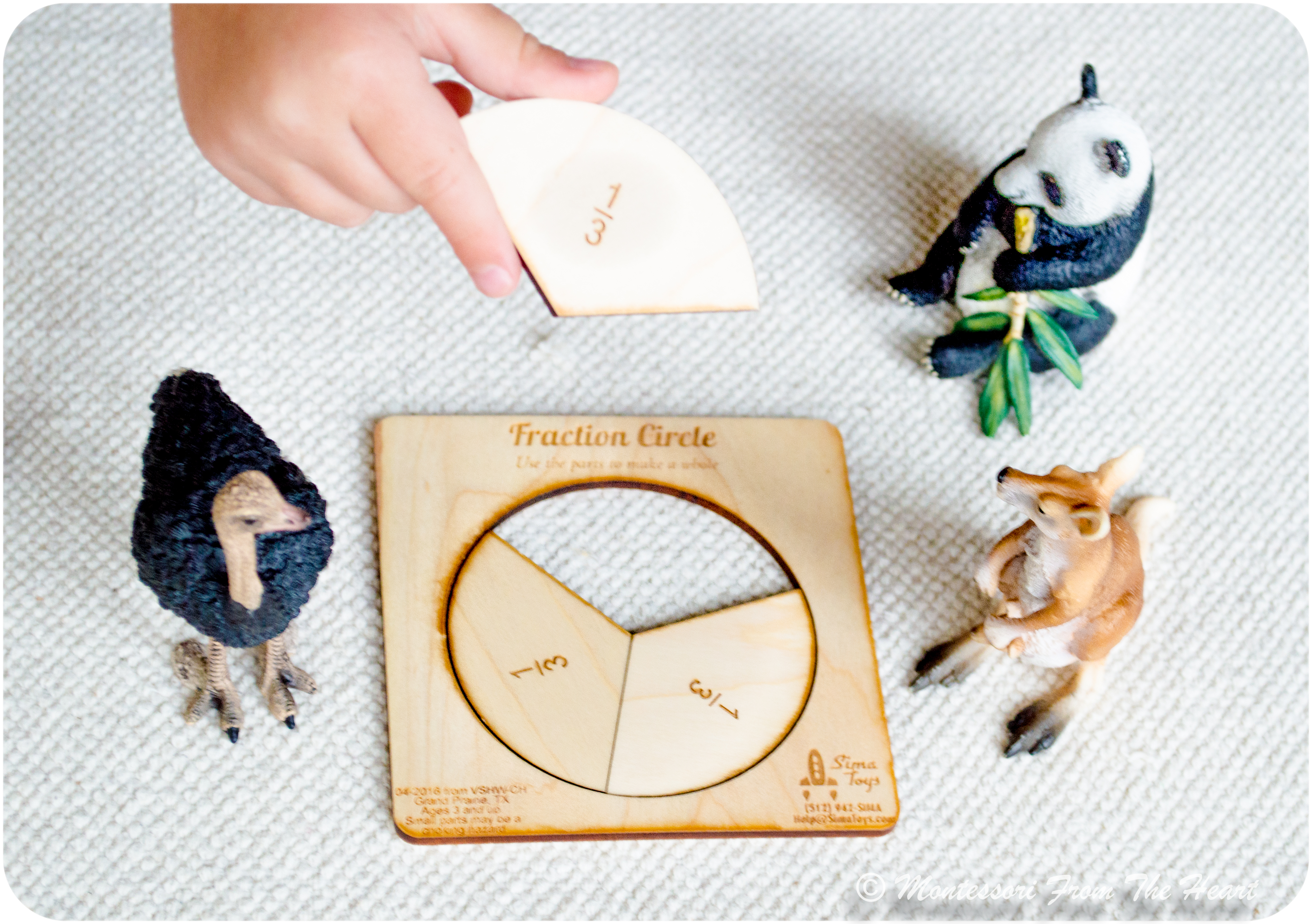Pick a 1/3 slice: “This says 1 cut into 3 pieces or one-third – 1/3." Have the child find the other two pieces that say 1/3.1/3

1 whole (top number/numerator) is "cut" into 3 pieces (bottom number/denominator which tells us how many pieces was the whole divided).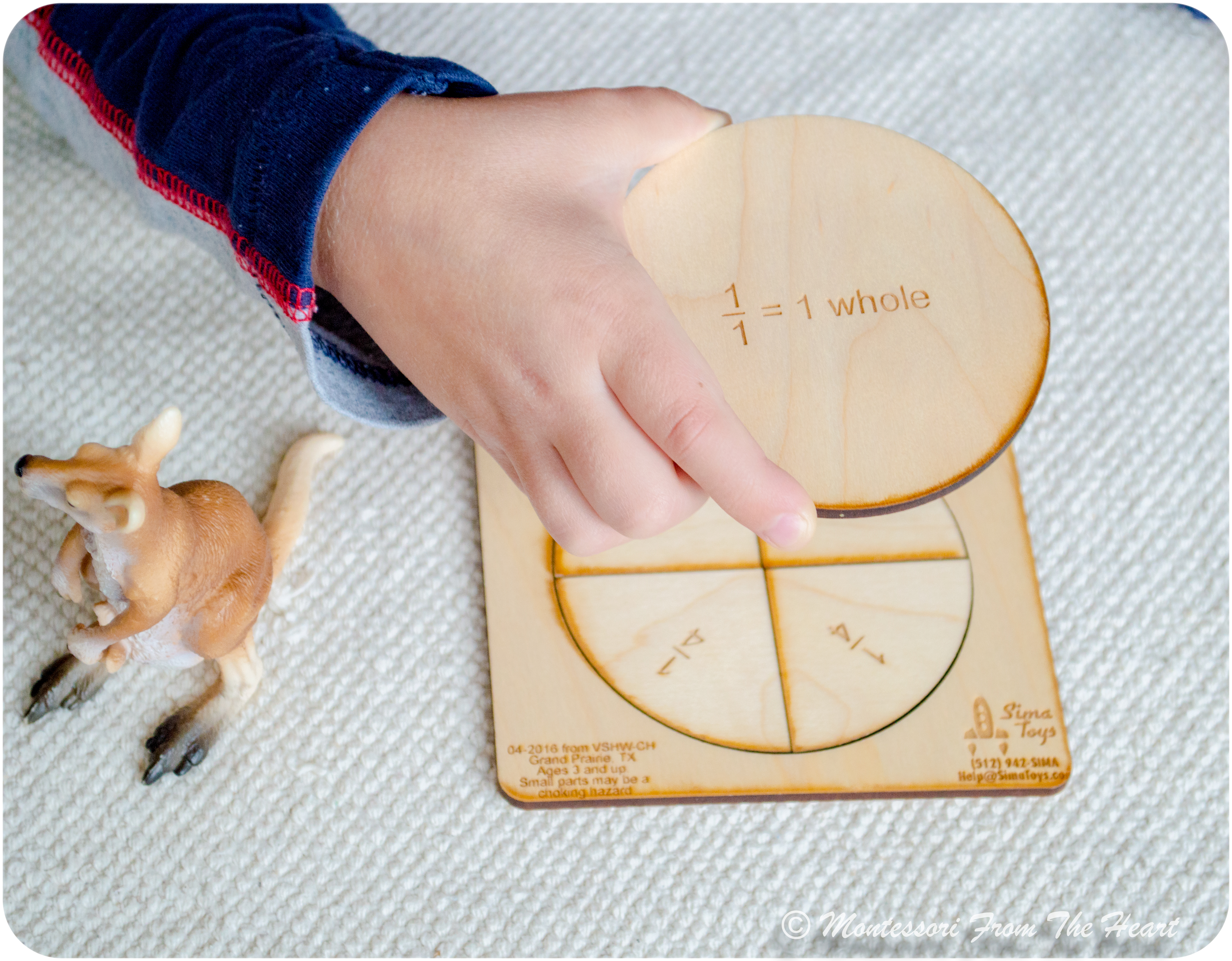Have the child place the whole non-cut pizza/circle on top of any fractioned circle.Now we have four animal friends – we need four slices (one for each).

"Let's find a piece that says 1 cut into 4 or 1/4 or a quarter. How many pieces do we need? The bottom number indicates the number of equal parts into which the unit/our pizza is divided." Have the child find all four quarters or 1/4.  "Is the pizza made out of quarters the same as a non-cut whole pizza?"  Have the child again place the whole pizza/circle on top of four-quartered pizza.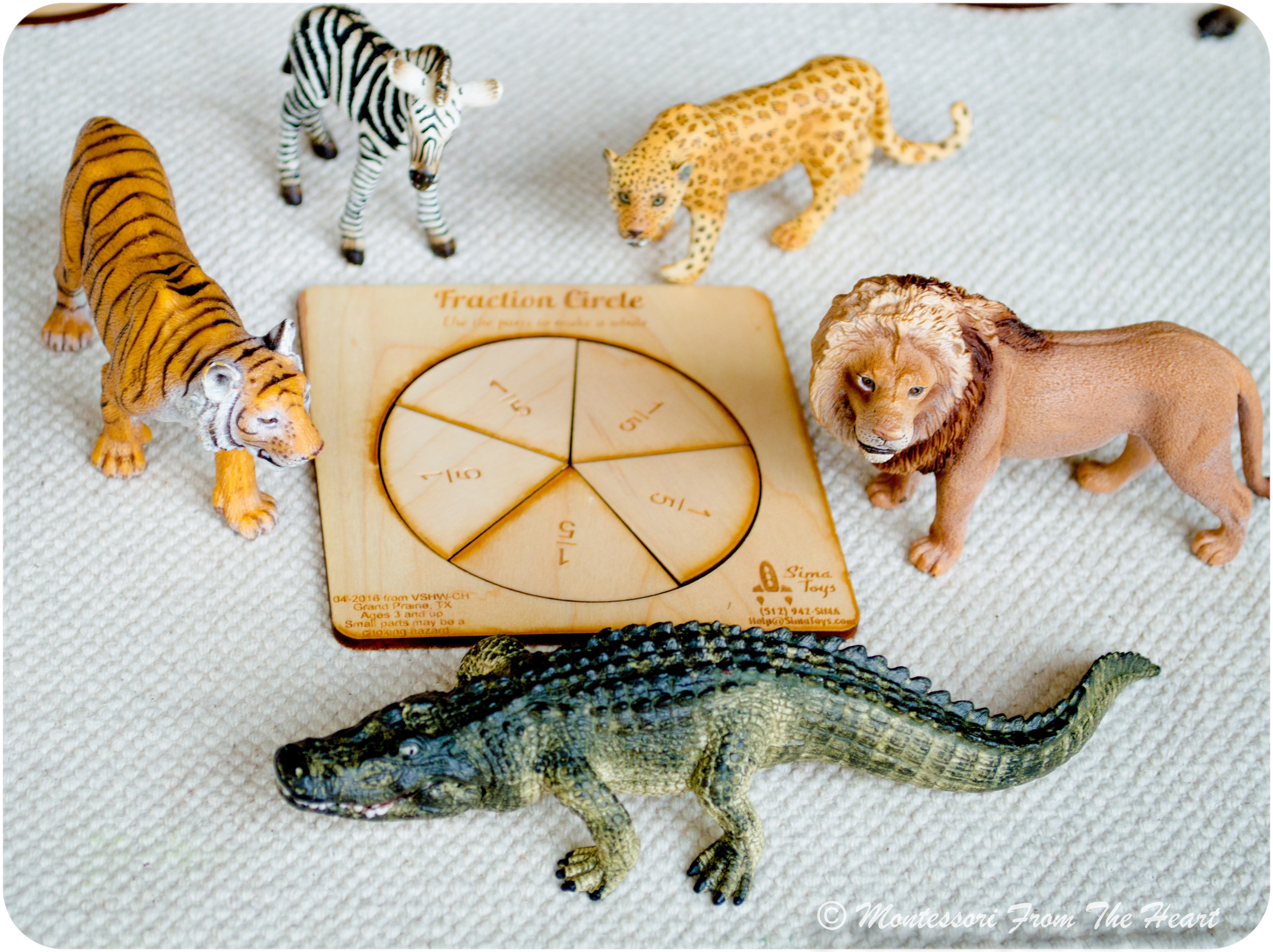Now, we have five friends hungry for pizza. Adrian noticed that the larger the bottom number, the smaller the pizza slice each friend is getting.You can also present a 3🅿️🌠 Three Period Lesson (see details here):

(P1) This is 1/3; (P2) Show me 1/3;  (P3) What is this?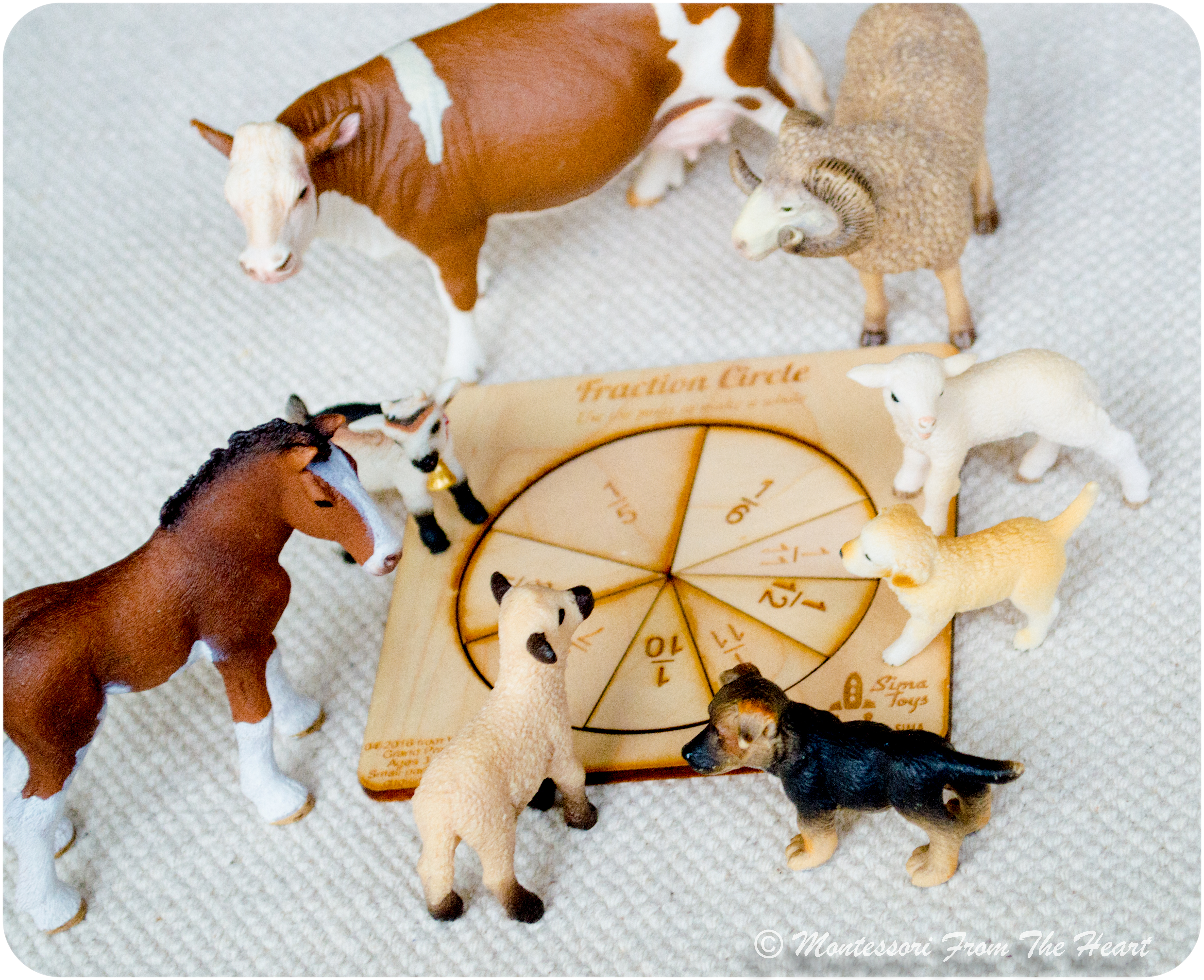As an extension, ask the child to build a "whole" from different fraction combinations.

Using fraction models, while demonstrating the written form next to it, visually illustrates the meaning of numerator and denominator. For example “ 1 over X”  or 1/X:

1 WHOLE (Pizza)/numerator

"over"/divide/cut pizza into…

X BOTTOM Number (denominator) tell us how many pieces the pizza is divided

Also, it is important to explain that any shape can be fractioned, not just a circle.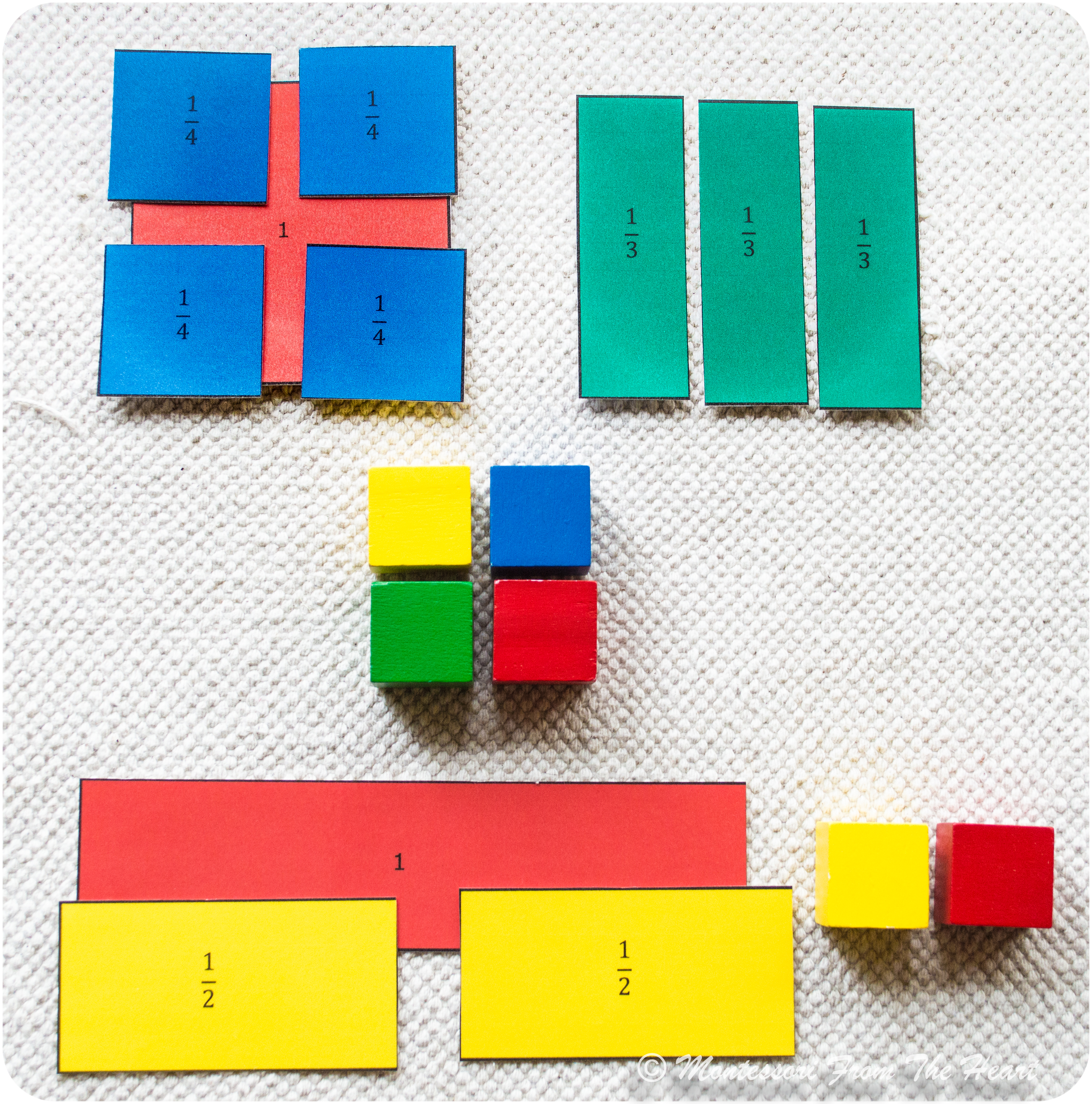Besides pizza, fractioning a "chocolate bar" which can be a rectangle or a square.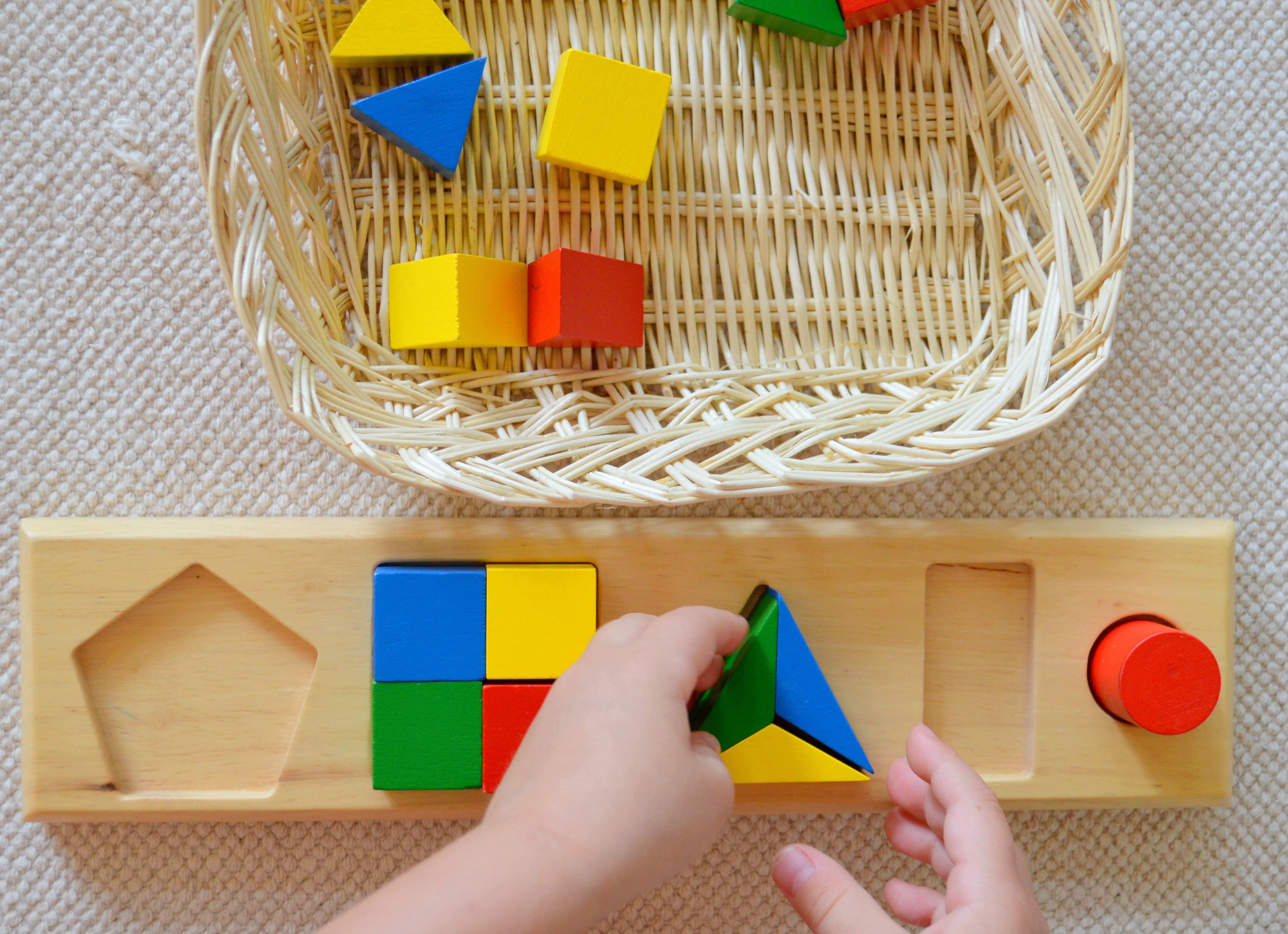This Montessori wooden Shape Fraction Sorter teaches a child shape, color and size recognition as well as early geometry by developing an understanding of geometric fractions.

Similar Montessori Wooden Shape Board/ Fraction Sorter (is available here), which fractions a circle, a triangle and a square. I also like this Geometric Puzzle, which introduces a child to shapes and fractions by having a written representation of fractions -whole, half and quarter – engraved onto the puzzle tray.Fractions in a pentagon shape were tricky, as pieces had to be rotated exactly to fit in.

Fraction models (whether cards or Montessori materials) can be used as a visual and tactile aid as the child moves into comparing and ordering fractions, adding and subtracting, as well as multiplying and dividing fractions. Through concrete representations, preferably while utilizing familiar objects such as animals figurines, a child will perceive learning as desirable and enjoyable. This was a fun learning through play experience, as Adrian really enjoyed cutting a pizza and feeding his animal friends!

For more on fractions, read a post "Learning Fractions with LEGO" here.